Steenrod-Eilenberg axioms

(Redirected from Steenrod–Eilenberg axioms)

Axioms describing the basic properties of homology (cohomology) groups (cf. Cohomology group; Homology group), which uniquely define the relevant homology (cohomology) theory. An axiomatic homology theory is defined on a certain category of pairs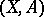of topological spaces if for any integeran Abelian group (or module over some ring)is assigned to every pair, while a homomorphism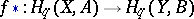is assigned to each mappingin such a way that the following axioms are satisfied:

1)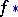is the identity isomorphism ifis the identity homeomorphism;

2), where;

3) connecting homomorphisms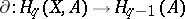are defined such that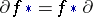(here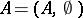,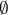is the empty set, while the mapping, induced by, is also denoted by);

4) the exactness axiom: The homology sequence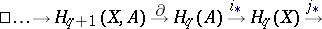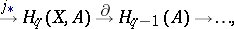where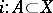,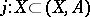are inclusions, is exact, i.e. the kernel of every homomorphism coincides with the image of the previous one;

5) the homotopy axiom: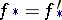for homotopic mappings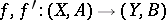in the category under consideration;

6) the excision axiom: If the closure inof an open subsetinis contained in the interior of, and the inclusion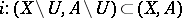belongs to the category, then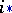is an isomorphism;

7) the dimension axiom: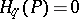when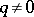for any singleton. The groupis usually called the coefficient group. Axiomatic cohomology theories are dually defined (homomorphismsare assigned to mappings; the connecting homomorphisms take the form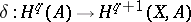). In the category of compact polyhedra, the ordinary homology and cohomology theories are the unique axiomatic theories with a given coefficient group (the uniqueness theorem). In the category of all polyhedra, the uniqueness theorem holds when the requirement is added that the homology (cohomology) of a union of open-closed, pairwise-disjoint subspaces be naturally isomorphic to the direct sum of the homology (direct product of the cohomology) of the subspaces (Milnor's additivity axiom). An axiomatic description of homology and cohomology theory also exists in more general categories of topological spaces (see , ). Generalized cohomology theories satisfy all the Steenrod–Eilenberg axioms (except for the dimension axiom), but are not uniquely defined by them.